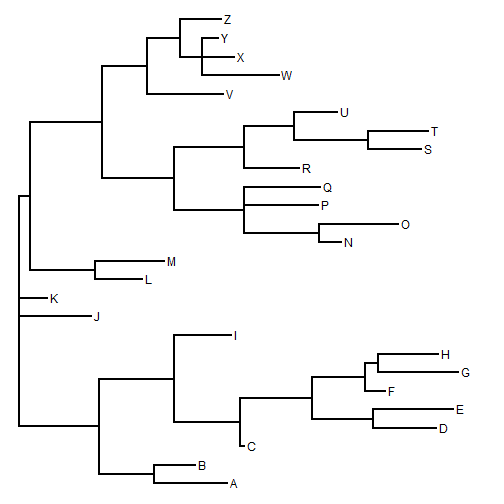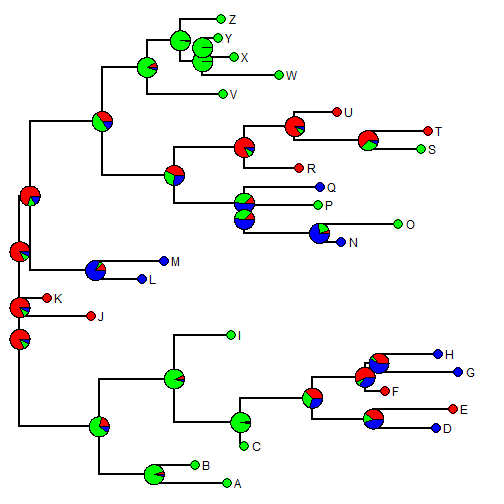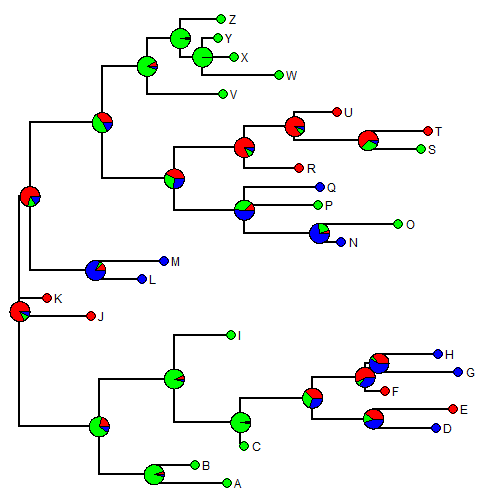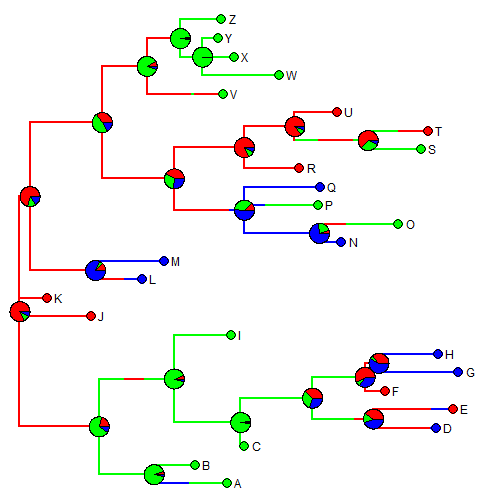## Tuesday, June 23, 2015

### Update to rerootingMethod for ancestral state reconstruction to permit polytomies

A phytools user recently reported that the ancestral character estimation method for discrete traits in phytools, `rerootingMethod`, does not work for trees with polytomies. This is in fact correct - although I had long forgotten that this was the case.

The function `rerootingMethod` uses the approach of Yang et al. (1995) to compute the marginal ancestral states by re-rooting the tree at all internal nodes. For instances in which some states at the tips are uncertain, it can also re-root at the tip to compute empirical Bayesian posterior probabilities for the leaves as well. The function is less relevant now that `ace` in the ape package also can compute the marginal ancestral states. (Although, as far as I know, `ace` can also not handle polytomies.) `rerootingMethod` stays somewhat relevant only by virtue of being able to compute posterior probabilities at tips, and for handling uncertainty at tip states (two sides of the same coin, I guess).

It was fairly straightforward to update `rerootingMethod` to handle polytomies, and the code is posted here. The way that this current version works is pretty simple. It just first takes the input tree, checks for polytomies using `is.binary.tree` in the ape package, resolves polytomies randomly with branches of zero length using `multi2di`, estimates ancestral states for all internal nodes (& tips, if `tips=TRUE`), and the uses the phytools function `matchNodes` to back-translate the reconstructed nodes in the resolved tree to the original, input tree. Note that although it is doing something here that `ace` does not do - the code internally uses (modified code from) `ace`.

Here - we can check it out by simulating a tree with some polytomies, generating trait data for tips & nodes, and then reconstructing ancestral states on the tree:

``````library(phytools)
dt<-rtree(n=26)
dt\$tip.label<-LETTERS
## set some of the internal branches
ii<-which(dt\$edge[,2]>Ntip(dt))
dt\$edge.length[sample(ii,4)]<-0
mt<-di2multi(dt)
plotTree(mt)
``````Next let's simulate a character up the tree:

``````Q<-matrix(c(-1,0.5,0.5,
0.5,-1,0.5,0.5,0.5,-1),3,3,
dimnames=list(letters[1:3],letters[1:3]))
st<-sim.history(mt,Q)
``````
``````## Done simulation(s).
``````
``````x<-st\$states
y<-getStates(st,"nodes")
``````

Now, we can reconstruct & plot ancestral states using `ace` on the bifurcating tree; and `rerootingMethod` on the bi- and on the multifurcating trees. If the methods are working properly then the reconstructions will be the same - although in the bifurcating node there will be additional nodes with states identical to other nodes from which they are separated by branches of zero length.

``````## first ace
fit1<-ace(x,mt,type="discrete",model="ER") ## doesn't work
``````
``````## Error in ace(x, mt, type = "discrete", model = "ER"): "phy" is not rooted AND fully dichotomous.
``````
``````fit2<-ace(x,dt,type="discrete",model="ER") ## doesn't work
``````
``````## Error in ace(x, dt, type = "discrete", model = "ER"): some branches have length zero or negative
``````
``````## set zero-length branches to be 1/1000000 total tree length
dst<-dt
dst\$edge.length[dst\$edge.length==0]<-max(nodeHeights(dt))*1e-6
fit3<-ace(x,dst,type="discrete",model="ER")
fit3
``````
``````##
##     Ancestral Character Estimation
##
## Call: ace(x = x, phy = dst, type = "discrete", model = "ER")
##
##     Log-likelihood: -25.3342
##
## Rate index matrix:
##   a b c
## a . 1 1
## b 1 . 1
## c 1 1 .
##
## Parameter estimates:
##  rate index estimate std-err
##           1   0.3278  0.1042
##
## Scaled likelihoods at the root (type '...\$lik.anc' to get them for all nodes):
##          a          b          c
## 0.82837257 0.09781827 0.07380916
``````

Now with the new version of `rerootingMethod`:

``````packageVersion("phytools")
``````
``````##  '0.4.57'
``````
``````fit4<-rerootingMethod(mt,x,model="ER")
fit4
``````
``````## \$loglik
##  -25.33421
##
## \$Q
##            a          b          c
## a -0.6556595  0.3278298  0.3278298
## b  0.3278298 -0.6556595  0.3278298
## c  0.3278298  0.3278298 -0.6556595
##
## \$marginal.anc
##               a          b            c
## 27 0.8283833959 0.09781145 0.0738051507
## 28 0.2121485528 0.69489845 0.0929529991
## 29 0.0532939602 0.91028552 0.0364205221
## 30 0.0627113681 0.88952034 0.0477682947
## 31 0.0132238730 0.97569370 0.0110824277
## 32 0.3870898035 0.30541903 0.3074911711
## 33 0.4035347684 0.15548939 0.4409758369
## 34 0.5865138808 0.07266794 0.3408181834
## 35 0.3530969369 0.06523786 0.5816652004
## 36 0.7045263050 0.13922658 0.1562471186
## 37 0.1288634178 0.06087131 0.8102652730
## 38 0.3401006710 0.49952777 0.1603715570
## 39 0.4129576403 0.32707964 0.2599627226
## 40 0.1266883464 0.35550347 0.5178081798
## 41 0.0553700550 0.21371716 0.7309127832
## 42 0.8088376946 0.10680265 0.0843596595
## 43 0.8570510600 0.08945954 0.0534894017
## 44 0.6274100032 0.30199393 0.0705960682
## 45 0.0836944308 0.86315981 0.0531457619
## 46 0.0119373448 0.97893201 0.0091306462
## 47 0.0006664523 0.99874487 0.0005886784
``````

If we plot both of these on our trees in turn, we should see that the marginal reconstructions (excepting the small deviation that was required to give our tree non-zero branch lengths throughout) should be equal:

``````## first ace
plotTree(dt,offset=0.5)
nodelabels(pie=fit3\$lik.anc)
tiplabels(pie=to.matrix(x,seq=letters[1:3]),cex=0.5)
````````````## now phytools
plotTree(mt,offset=0.5)
nodelabels(pie=fit4\$marginal.anc)
tiplabels(pie=to.matrix(x,seq=letters[1:3]),cex=0.5)
``````Finally, for fun let's overlay the true, known states to see how close (or off) we were:

``````plotSimmap(st,colors=setNames(c("red","green","blue"),letters[1:3]),
offset=0.5)
nodelabels(pie=fit4\$marginal.anc)
tiplabels(pie=to.matrix(x,seq=letters[1:3]),cex=0.5)
``````That's it.

1.I am unable to run the rerootingMethod, despite it having the same format input of 'x':

Error in `colnames<-`(`*tmp*`, value = c("a", "c")) :
attempt to set 'colnames' on an object with less than two dimensions

Any suggestions? Thanks

1.Hi Alex. What is your object x? It should be a vector of type character (or a factor) with names corresponding to the species names in the tree.

2.Hi Liam,
I’m working on adding evolution of a discrete character to a phylogeny. First I am attempting to model ER, SYM, and ARD using rerootingMethod. ER and ARD seem to work properly, with ARD being a better fit. SYM, however, produces the error “In log(comp[1:M + N]) : NaNs produced”. I suspect from your post here (http://blog.phytools.org/2016/05/more-on-plot-method-for-object-class.html) it has to do with parameters versus tips, but in that case, why does ARD seem to work? Furthermore, when I move onto using make.simmap to see the (code: SYMtrees <- make.simmap(tree,character,model="SYM",nsim=1000,q="mcmc"), the resulting sectors in the node piecharts are all equal, and the same process for ARD produces NaNs but with a sensible output. Does this suggest that the character and phylogeny will only support the simpler ER model? Is there something else I am missing? Thank you!
-Bob

3.dst<-tree
> dst\$edge.length[dst\$edge.length==0]<-max(nodeHeights(tree))

How can this be used on a class of multi.phylo (I have over 3000 posterior trees from a Bayes anaylsis).

I am trying to run SIMMAP but it keeps giving me this error " Error in xx[tree\$tip.label, ] : subscript out of bounds"

I assume that means I need to use the code you used above to set non zero lengths to 1/1000 however when I try to use the code above I get this error " Error in nodeHeights(ntrees) : tree should be an object of class "phylo"."

However, When I use 'is.binary.multiphylo' I get "true" for all 3000 trees. Any help with this would be greatly appreciated.

4.This comment has been removed by the author.

5.Hi, just wondering how nodes are named. I've used this method for >100 discrete characters and now want to investigate each node, for each character. How do I know which node "155" refers to?

Note: due to the very large amount of spam, all comments are now automatically submitted for moderation.